# iOS设计模式（代码分析系列2：简单工厂模式）

1、简述

2、定义

“专门定义一个类来负责创建其他类的实例，被创建的实例通常具有共同的父类。”

3、结构图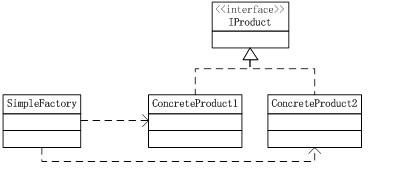ConcreteProduct1和ConcreteProduct2两个产品具有一个共同的父类IProject，简单工厂类为SimpleFactory，负责根据传入的不同参数来决定生产ConcreteProduct1还是ConcreteProduct2产品。

4、代码示例讲解

（1）除运算示例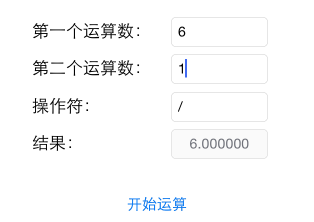（2）减运算示例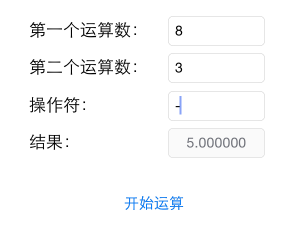?

 1 2 3 4 5 6 7 8 9 10 11 12 13 14 15 16 17 18 19 20 21 22 23 24 25 26 27 28 29 30 31 32 `- (IBAction)getResult:(id)sender {` `    ` `//得到三个文本输入框的内容` `    ` `NSString* strFirstNum = self.FirstNumTextField.text;` `    ` `NSString* strSecondNum = self.SecondNumTextField.text;` `    ` `NSString* strOperation = self.OperationTextField.text;` `    ` `//进行运算操作` `    ` `if`  `([strOperation isEqualToString:@` `"+"` `]) {` `        ` `NSLog(@` `"+"` `);` `        ` `double`  `result = [strFirstNum doubleValue]+[strSecondNum doubleValue];` `        ` `self.ResultTextField.text = [NSString stringWithFormat:@` `"%f"` `,result];` `    ` `}` `else`  `if` `([strOperation isEqualToString:@` `"-"` `]){` `        ` `NSLog(@` `"-"` `);` `        ` `double`  `result = [strFirstNum doubleValue]-[strSecondNum doubleValue];` `        ` `self.ResultTextField.text = [NSString stringWithFormat:@` `"%f"` `,result];` `    ` `}` `else`  `if` `([strOperation isEqualToString:@` `"*"` `]){` `        ` `NSLog(@` `"*"` `);` `        ` `double`  `result = [strFirstNum doubleValue]*[strSecondNum doubleValue];` `        ` `self.ResultTextField.text = [NSString stringWithFormat:@` `"%f"` `,result];` `    ` `}` `    ` `else`  `if` `([strOperation isEqualToString:@` `"/"` `]){` `        ` `NSLog(@` `"/"` `);` `        ` `//判断除数不能为0` `        ` `if`  `([strSecondNum isEqualToString:@` `"0"` `]) {` `            ` `NSLog(@` `"除数不能为0"` `);` `            ` `UIAlertView* tempAlert = [[UIAlertView alloc] initWithTitle:@` `"警告"`  `message:@` `"除数不能为0"`  `delegate:nil cancelButtonTitle:@` `"取消"`  `otherButtonTitles:nil];` `            ` `[tempAlert show];` `        ` `}` `else` `{` `            ` `double`  `result = [strFirstNum doubleValue]/[strSecondNum doubleValue];` `            ` `self.ResultTextField.text = [NSString stringWithFormat:@` `"%f"` `,result];` `        ` `}` `    ` `}` `}`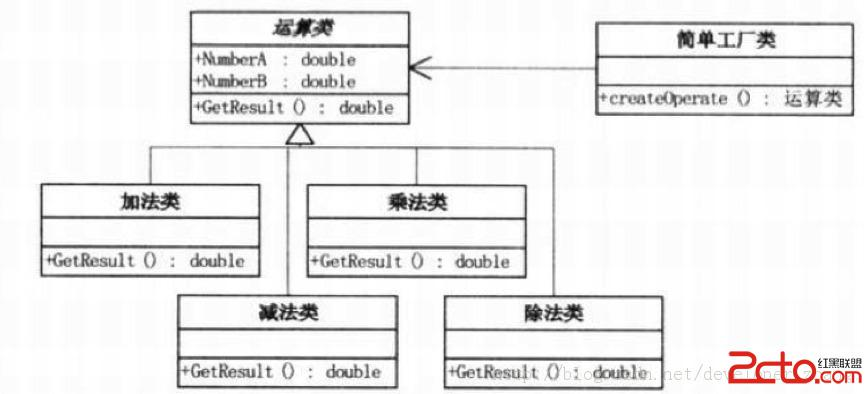（1）创建一个协议OprationProtocol，由父类Operation来遵从该协议

?

 1 2 3 4 5 6 `/*` ` ` `*  操作方法协议接口` ` ` `*/` `@protocol OperationProtocol ` `-(` `double` `)getResult;` `@end `

（2）定义加减乘除操作的父类Operation

?

 1 2 3 4 5 6 7 8 `#import OperationProtocol.h` `/*` ` ` `*  操作方法父类` ` ` `*/` `@interface Operation : NSObject` `@property ` `double`  `firstNum;` `//第一个操作数` `@property ` `double`  `secondNum;` `//第二个操作数 ` `@end`

（3）加减乘除实现类，此处以"加"举例说明，

?

 1 2 3 4 5 6 7 8 9 10 11 12 13 14 15 16 17 18 `//OperationAdd.h文件` `#import Operation.h` `/*` ` ` `*  加法实现类` ` ` `*/` `@interface OperationAdd : Operation` `@end`   `//OperationAdd.m文件` `#import "OperationAdd.h"` `@implementation OperationAdd` `-(` `double` `)getResult` `{` `    ` `double`  `result = 0;` `    ` `result = self.firstNum+self.secondNum;` `//"+"是OperationAdd时候使用，"+-*/"分别对应"加减乘除"` `    ` `return`  `result;` `}` `@end`

（4）简单工厂类的代码，

?

 1 2 3 4 5 6 7 8 9 10 11 12 13 14 15 16 17 18 19 20 21 22 23 24 25 26 27 28 29 30 31 32 33 34 35 36 `//OpeartionFactory.h file` `#import Operation.h` `#import OperationAdd.h` `#import OperationSub.h` `#import OperationMultiply.h` `#import OperationDivide.h` `/*` ` ` `*  操作工厂类` ` ` `*/` `@interface OperationFactory : NSObject` ` `  `//获得操作对象` `+(Operation*)createOperate:(NSString*)operateStr;` ` `  `@end`   `//OpeartionFactory.m file` `#import "OperationFactory.h"` `@implementation OperationFactory`   `+(Operation*)createOperate:(NSString*)operateStr` `{` `    ` `Operation* oper = nil;` `    ` `//根据不同的操作符，创建不同的操作对象，"+-*/"分别对应"加减乘除"` `    ` `if`  `([operateStr isEqualToString:@` `"+"` `]) {` `        ` `oper = [[OperationAdd alloc] init];` `    ` `}` `else`  `if`  `([operateStr isEqualToString:@` `"-"` `]){` `        ` `oper = [[OperationSub alloc] init];` `    ` `}` `else`  `if`  `([operateStr isEqualToString:@` `"*"` `]){` `        ` `oper = [[OperationMultiply alloc] init];` `    ` `}` `else`  `if`  `([operateStr isEqualToString:@` `"/"` `]){` `        ` `oper = [[OperationDivide alloc] init];` `    ` `}` `    ` `return`  `oper;` `}` `@end`

（5）客户端代码，在ViewController中使用OperationFactory

?

 1 2 3 4 5 6 7 8 9 `- (IBAction)clickingOperation:(id)sender {` `    ` `NSString* strFirstNum = self.firstNumTextField.text;` `    ` `NSString* strSecondNum = self.secondNumTextField.text;` `    ` `Operation* oper;` `    ` `oper = [OperationFactory createOperate:self.operationTextField.text];` `    ` `oper.firstNum = [strFirstNum doubleValue];` `    ` `oper.secondNum = [strSecondNum doubleValue];` `    ` `self.resultTextField.text = [NSString stringWithFormat:@%f,[oper getResult]];` `}`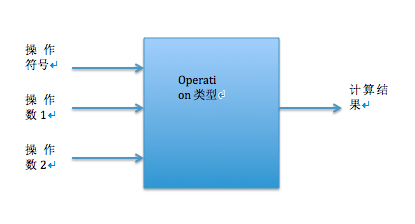（1）工厂类负责创建的对象较少时；

（2）客户端只知道传入工厂类的参数，对于如何创建对象的逻辑不必关心时。### 法斗斗#### 暂无文章

CentOS7.6中安装使用fcitx框架

5
0
《Designing.Data-Intensive.Applications》笔记 四

8
0
docker 使用mysql

1， 进入容器 比如 myslq1 里面进行操作 docker exec -it mysql1 /bin/bash 2. 退出 容器 交互： exit 3. mysql 启动在容器里面，并且 可以本地连接mysql docker run --name mysql1 --env MY...

10
0
python数据结构

1、字符串及其方法（案例来自Python-100-Days） def main(): str1 = 'hello, world!' # 通过len函数计算字符串的长度 print(len(str1)) # 13 # 获得字符串首字母大写的...

huijue

6
0
PHP+Ajax微信手机端九宫格抽奖实例

PHP+Ajax结合lottery.js制作的一款微信手机端九宫格抽奖实例，抽奖完成后有收货地址添加表单出现。支持可以设置中奖概率等。 奖品列表 <div class="lottery_list clearfix" id="lottery"> ......

ymkjs1990

5
0